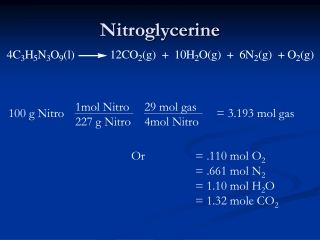DownloadDownload PresentationNitroglycerine

# Nitroglycerine

Télécharger la présentation## Nitroglycerine

- - - - - - - - - - - - - - - - - - - - - - - - - - - E N D - - - - - - - - - - - - - - - - - - - - - - - - - - -
##### Presentation Transcript

1. 1mol Nitro 227 g Nitro 29 mol gas 4mol Nitro Nitroglycerine 100 g Nitro = 3.193 mol gas Or = .110 mol O2 = .661 mol N2 = 1.10 mol H2O = 1.32 mole CO2

2. 1atm Nitroglycerine V = nRT/P PV = nRT 3.193 mol 425 C 698 K (0.082057 L atm/mol K) V = V = 183 L

3. FW Balanced Eq Moles Cl2 Moles HCl Select limiting reagent FW Balanced Eq Moles H2 Moles HCl H2 + Cl2 2HCl(g) Limiting Reactant Problem – the hard way Final pressure = pressure of HCl + pressure of excess reagent g of Cl2 g of H2 • Starting moles H2 • Moles H2 used • Excess moles H2 Moles Cl2 Moles H2used PV = nRT + Moles HCl Moles H2xs Total moles of gas P = 193 torr

4. FW PV = nRT Moles Cl2 Pressure Cl2 Add to get Initial pressure FW PV = nRT Moles H2 Pressure H2 H2 + Cl2 2HCl(g) Understanding Gases – the easy way Remember that the composition of a gas does NOT effect the pressure it exerts. So, since there is no change in the number of MOLES of gas during the reaction, there will be no change in the pressure. Thus the initial pressure equals the final pressure. Final pressure = Initial P = P Cl2 + P H2 g of Cl2 g of H2

5. g of A g of C FW FW Molarity Moles C Molarity Volume of A Volume of C Volume Volume Molarity of A Molarity of C # grams # grams Molecular weight Of A Molecular weight Stoichiometry + A B C + D Balanced Eq Moles A

6. Pressure of A Pressure of C Balanced Eq (T and P/V same) Volume of A Volume of C PV = nRT PV = nRT FW g of C Molarity Moles C Molarity Volume of A Volume of C Volume Volume Molarity of A Molarity of C # grams # grams Molecular weight Of A Molecular weight Gas Stoichiometry + A B C + D Gases only!!!! g of A FW Balanced Eq Moles A

7. What is RMS for H2 at room temp? = 1kg _ 1000g 2.0g/mol = 1.92 x 103 m/s ≈ 2000 m/s ≈ 4300 mi/hr

8. Problems involving Gases • PV=nRT can be used to get moles of gas from P, V and T. Once you have mole then it is just a normal limiting reagent or stoichiometry or titration or ….

9. Gas Law Shortcuts • All revolve around the fact that in gases, V and P does NOT depend on nature of gas. • Implications • Can sum moles of all products and then find P or V. (Dalton’s Law of Partial Pressures.) • Ratio of n:V or n:P is constant (at a given temp and P or V) So 2 moles of gas C takes up twice the volume of 1 mole of gas A at the same conditions. • Caution: Shortcuts are NOT always present.

10. Polarity and IMF • Polarity is the key to IMFs. • Select 2 molecules involved, find polarity of each. • Polar/polar = dipole/dipole -strongest • Nonpolar/nonpolar = induced/induced - strongest • Polar / Nonpolar = dipole induced-dipole -strongest • If dipole/dipole look for H-Bonding • Requires:

11. Why don’t oil and water mix?